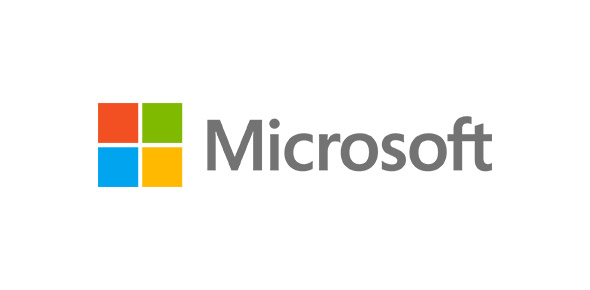# What Is Cryptographic Service Quiz?

26 Questions | Total Attempts: 45SettingsThis is a quiz for all the computer lovers out there. If you want to test your knowledge on this particular bunch of random IT facts take this quiz and find out how vast your knowledge is on cryptographic service.

• 1.
Steganography can use image files, audio files, or even video files to contain hidden information.
• A.

True

• B.

False

• 2.
A hash that is created from a set of data can be reversed.
• A.

True

• B.

False

• 3.
Cryptography cannot be applied to entire disks.
• A.

True

• B.

False

• 4.
Self-encrypting HDD is commonly found in copiers and multifunction printers as well as point-of-sale systems used in government, financial, and medical environments.
• A.

True

• B.

False

• 5.
Most HSMs are PAN-based appliances that can provide services to multiple devices.
• A.

True

• B.

False

• 6.
One of the most famous ancient cryptographers was ____.
• A.

Albert Einstein

• B.

Isaac Newton

• C.

Julius Caesar

• D.

Caesar Augustus

• 7.
____ is text that has no formatting (such as bolding or underlining) applied.
• A.

Plaintext

• B.

Plain text

• C.

Simpletext

• D.

Simple text

• 8.
ensures that the information is correct and no unauthorized person or malicious software has altered that data.
• A.

Confidentiality

• B.

Availability

• C.

Encryption

• D.

Integrity

• 9.
The most basic type of cryptographic algorithm is a ____ algorithm.
• A.

Hash

• B.

Key

• C.

Digest

• D.

Block

• 10.
____ takes plaintext of any length and creates a hash 128 bits long.
• A.

RSA

• B.

SHA1

• C.

MD5

• D.

MD2

• 11.
In MD5, the length of a message is padded to ____ bits.
• A.

32

• B.

64

• C.

128

• D.

512

• 12.
____ is a relatively recent cryptographic hash function that has received international recognition and adoption by standards organizations, including the International Organization for Standardization (ISO).
• A.

Twofish

• B.

Blowfish

• C.

Whirlpool

• D.

Rijndal

• 13.
Symmetric encryption is also called ____ cryptography.
• A.

Private key

• B.

Public key

• C.

Symmetric key

• D.

Shared key

• 14.
The simplest type of stream cipher is a ____ cipher.
• A.

Shift

• B.

Substitution

• C.

Lock

• D.

Loop

• 15.
A ____ cipher rearranges letters without changing them.
• A.

Substitution

• B.

Block

• C.

Loop

• D.

Transposition

• 16.
____ is designed to replace DES.
• A.

AES

• B.

Twofish

• C.

3DES

• D.

RSA

• 17.
The____ is a symmetric cipher that was approved by the NIST in late 2000 as a replacement for DES.
• A.

AES

• B.

3DES

• C.

RSA

• D.

Twofish

• 18.
The algorithm ____ is a block cipher that operates on 64-bit blocks and can have a key length from 32 to 448 bits.
• A.

RSA

• B.

AES

• C.

Blowfish

• D.

3DES

• 19.
Encryption uses two keys instead of only one and these keys are mathematically related.
• A.

Symmetric

• B.

Asymmetric

• C.

Shared

• D.

Public key

• 20.
The basis for a digital signature rests on the ability of ____ keys to work in both directions.
• A.

Symmetric

• B.

Shared

• C.

Unique

• D.

Asymmetric

• 21.
The ____ algorithm is the most common asymmetric cryptography algorithm and is the basis for several products.
• A.

AES

• B.

RSA

• C.

Twofish

• D.

Blowfish

• 22.
A ____ is a number divisible only by itself and 1.
• A.

Prime number

• B.

Prime decimal

• C.

compound number

• D.

Neutral number

• 23.
was first proposed in the mid-1980s and it uses sloping curves.
• A.

FCC

• B.

RSA

• C.

ECC

• D.

IKE

• 24.
____ attempts to use the unusual and unique behavior of microscopic objects to enable users to securely develop and share keys as well as to detect eavesdropping.
• A.

Reactive cryptography

• B.

Symmetric cryptography

• C.

Analog cryptography

• D.

Quantum cryptography

• 25.
NTRUEncrypt uses ____ cryptography that relies on a set of points in space.
• A.

Matrix-based

• B.

Lattice-based

• C.

Linear

• D.

Quantum

Related TopicsBack to top